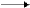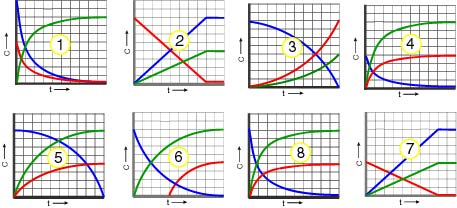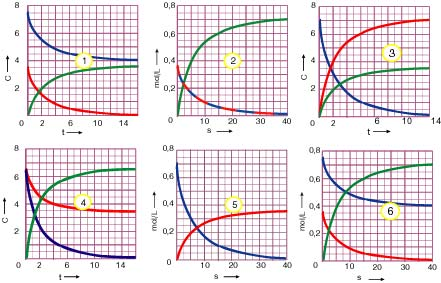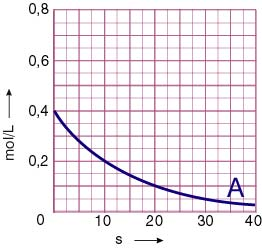EXERCISES

1.   Calculate the order of each one of the following reactions and the units of the rate constant, if we assume that the reactions are simple (take place in one step).
α) ΑP                 β) Α + ΒP                  γ) Α + 2ΒP
where P: products
Solution

2.   The reaction,  Α + 2ΒP,  is simple (one step) and it takes place in an aquatic solution. Examine the rate change in each one of the following cases:

a) If the concentration of A is doubled

b) If the concentration of B is doubled

c) If we dilute the solution with dionised water until its original volume is doubled.                                                               Solution

3. In a 250ml solution of HCl we add Zn and the following reaction takes place:  Zn + 2HClZnCl2 + H2. After the elapse of 80 seconds since initiation, 112ml of H2 have been produced calculated in STP. Estimate the mean reaction rate at the first 80 seconds.

Solution

4.For the reaction 2Α + Βthe following experimental data was gathered.

 sec [A] mol/L .a) Estimate the mean reaction rate for the periods 0-10 sec and 10-20 sec. b) Estimate the order of the reaction. 0 10 20 30 1.5 1.0 0.5 0.0

5.For the reaction 2Α + ΒC,  the following experimental data was gathered.

 s [Α] mol/L [Β] mol/L α) Estimate the mean reaction rate for the periods 0-10 sec and 0-30 sec. β) Represent graphically the changes of A, B and C.                                 Answer 0 10 30 60 0.80 0.30 0.14 0.04 0.50 0.25 0.17 0.12

6.  Which one of the following diagrams corresponds to the simple reaction
2Α2Β + C ;7. In a closed vessel the gases A and B are introduced with the moles ratio 1:2. The vessel is heated at a spesific tepmerature at which A and B react  in quantity according to the chemical equation:
Α + 2Β2Γ. Draw the diagrams of the changes of A, B and C if it is known that the reaction is NOT zero order.
Solutionη

8.For the reaction  2ΑΒ,   the following experimental data was gathered:

 s 0 10 20 30 [Α] mol/L 1 0.75 0.5 0.25

α)Represent  grapfhically C versus time in sec of A and B.
β) If the rate of the reaction, 30 seconds after initiation, is 0.007 mol/L.s, calculate the rate at the begging of the reaction.

9. In each one of the following reactions
a) A + B2C  same molar concentration of Α and Β.
b) A + B2C  2a mol of A and a mol of Β.
c) 2A2B + C
d) 2AB
corresponds one of the following diagrams:Two of these diagrams don't have a match. Find the correspondance between the reactions and the diagrams.

10.  In a closed vessel we introduce 0,4 moles of gas A, which dissociate at a particular temperature according to the chemical equation Α2Β + Γ . From the study of the reaction the following experimental data was gathered for reactant A as shown in the table and the correspondent diagram.

 t (s) [Α] mol/L0 10 20 30 40 0.400 0.200 0.100 0.050 0,025

a) Calculate the mean reaction rate for the  periods 0-10 s, 10-20s, 20-30 s, 30-40 and 0-40 s.
b)
Complete the diagram by drawing the lines of B and C.

11.
In a vessel of volume 8L we introduce 0.3 mol of A and 0.2 mole of B, which at 127°C react according to the chemical reaction: Α + ΒΓ.  All the components of the system are gases. After the elapse of 50 seconds the pressure in the internal of the vessel becomes 1.64 atm.
a)
Calculate the moles of the gases after the elapse of 50 sec.
b) Calculate the moles of the gases after the elapse of 50 sec.
Solution

12. In a vessel of volume 2.5 L, we introduce 0.6 moles of gas A and 0.9 moles of gas B, the reaction that takes place is: Α + 3Β2C.

After the elapse of 50 sec from the start of the reaction, the rate is: 1.6.10 -4 mol/Ls.
a)
Calculate the decomposition rate of A and B, as well as the formation rate of C.

b) Find the composition of the mixture after the elapse of the 50 seconds.

13.  For the reaction Α + 3Β(where P: products) the following measurements took place with the corresponding results.

 a/a [Α] mol/L [Β] mol/L Rate  mol/L.s 1 0.1 0.1 4·10-3 2 0.2 0.1 8·10-3 3 0.1 0.2 1.6·10-2

α) Write down the rate low of the reaction
β) Determine the order of the reaction
γ) Find the arithmetical value and the units of the rate constant.
Solution

14.  For the reaction  aΑ + bΒP1 +P2  the following measurements took place with the corresponding results as shown in the table:

 a/a [Α] mol/L [Β] mol/L mol/L·s 1 0.2 0.2 1.2·10-3 2 0.1 0.2 6·10-4 3 0.2 0.6 1.08·10-2

a) Write down the rate low of the reaction

b) Determine the order of the reaction

c) Find the arithmetical value and the units of the rate constant of the reaction.

d) Find the initial rate of the reaction when it starts off with 0.4 moles of A and 1 mole of B in a vessel of volume 2L, when all of the reactants are gases.

15  For the reaction,  aΑ + bB + cCP1 + P the following measurements took place with the corresponding results as shown in the table.

.

 a/a [Α] mol/L [B] mol/L [C] mol/L υ mol/Ls 1 0.1 0.1 0.1 9·10-4 2 0.1 0.2 0.1 36.10-4 3 0.3 0.1 0.1 2710-4 4 0.1 0.2 0.2 7210-4

a) Write down the rate low of the reaction

b) Determine the order of the reaction

c) Find the arithmetical value and the units of the reaction’s constant.

16.
For the reaction  2Α + Βwhere A, B and P are gases,  the following measurements took place with the corresponding results as shown in the table.

 a/a Ρa atm Pb atm Rate  mol/L·s 1 0.2 0.2 1.2510-3 2 0.4 0.1 2.510-3 3 0.4 0.2 510-3

Write down the rate low, determine the order of the reaction and find the value of the rate low constant.

Solution

17.
For the reaction aΑ +bΒP, where A, B and P are gases, , the following measurements took place with the corresponding results as shown in the table.

 α/α ΡΑ atm PΒ atm Rate mole/Ls 1 0.3 0.6 910-4 2 0.1 0.3 1.510-4 3 0.15 0.2 1.510-4

a) Write down the rate low of the chemical reaction

b) Find the order of the reaction

c) Find the arithmetical value and the dimensions of the rate low constant.

18.   Gas A dissociates into gases B and C according to the reaction
ΑΒ + C.
If the arithmetical value of the rate equals the arithmetical value of the rate constant and under the condition that the concentration of A is not 1mol/L, estimate: a) the order of the reaction b) the dimensions of the constant.                                                      Answer

19.   In a vessel of volume 2 L we mix 0.6 mol NO and 0.4 mol O2. We heat the vessel at a specific temperature at which the initial rate of the reaction
2NO + O
22NO2    is  υ = 3,610-3 molL-1s-1. Calculate the rate of the reaction at the time that in the vessel exist 0.4 mol NO2, and take into consideration that the reaction is simple (only one step).

Solution

20.  In a vessel of 3 L we introduce 0.9 mol of gas A and 0.9 mol of gas B, which react at a particular temperature to give compound C according to the reaction
Α + Β2C.
The reaction takes place in one step. Calculate the moles of the gases that exist in the vessel, when the rate of the reaction is decreased to one fourth its initial value.

21. In a vessel of volume 3 L we introduce 6.4gr of gas A, which has molecular mass 80. The vessel is heated at 327°C and A dissociates according to the simple reaction2Β + C. After the elapse of 10 sec from the initiation of the reaction, the pressure in the internal of the vessel becomes 1.64 atm and after the elapse of 60 sec the pressure becomes 1.804 atm.